# How to Calculate and Solve for the Molar Concentration, Molar Mass and Mass Concentration in Chemistry | The Calculator Encyclopedia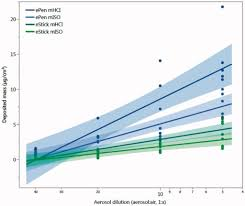The image above represents the mass concentration.

To compute for mass concentration, two essential parameters are needed and these are molar concentration (c) and molar mass (M).

ρ = c x M

Where;
ρ = mass concentration
c = molar concentration
M = molar mass

Let’s solve an example;
Find the mass concentration when the molar concentration is 24 and molar mass is 15.

This implies that;
c = molar concentration = 24
M = molar mass = 15

ρ = c x M
ρ = 24 x 15
ρ = 360

Therefore, the mass concentration is 360 Kg/dm³.

Calculating the Molar Concentration when the Mass concentration and Molar Mass.

c = ρ / M

Where;
c = molar concentration
ρ = mass concentration
M = molar mass

Let’s solve an example;
Find the molar concentration when the mass concentration is 120 with a molar mass of 40.

This implies that;
ρ = mass concentration = 120
M = molar mass = 40

c = ρ / M
c = 120 / 40
c = 3

Therefore, the molar concentration is 3 mol/L.

Calculating the Molar Mass when the Mass concentration and Molar Concentration .

M = ρ / c

Where;
M = molar mass
ρ = mass concentration
c = molar concentration

Let’s solve an example;
Find the molar mass when the mass concentration is 150 with a molar concentration of 30.

This implies that;
ρ = mass concentration = 150
c = molar concentration = 30

M = ρ / c
M = 150 / 30
M = 5

Therefore, the molar mass is 5 mol.

Nickzom Calculator – The Calculator Encyclopedia is capable of calculating the mass concentration.

To get the answer and workings of the mass concentration using the Nickzom Calculator – The Calculator Encyclopedia. First, you need to obtain the app.

You can get this app via any of these means:

To get access to the professional version via web, you need to register and subscribe for NGN 1,500 per annum to have utter access to all functionalities.
You can also try the demo version via https://www.nickzom.org/calculator

Once, you have obtained the calculator encyclopedia app, proceed to the Calculator Map, then click on Basic Chemistry under Chemistry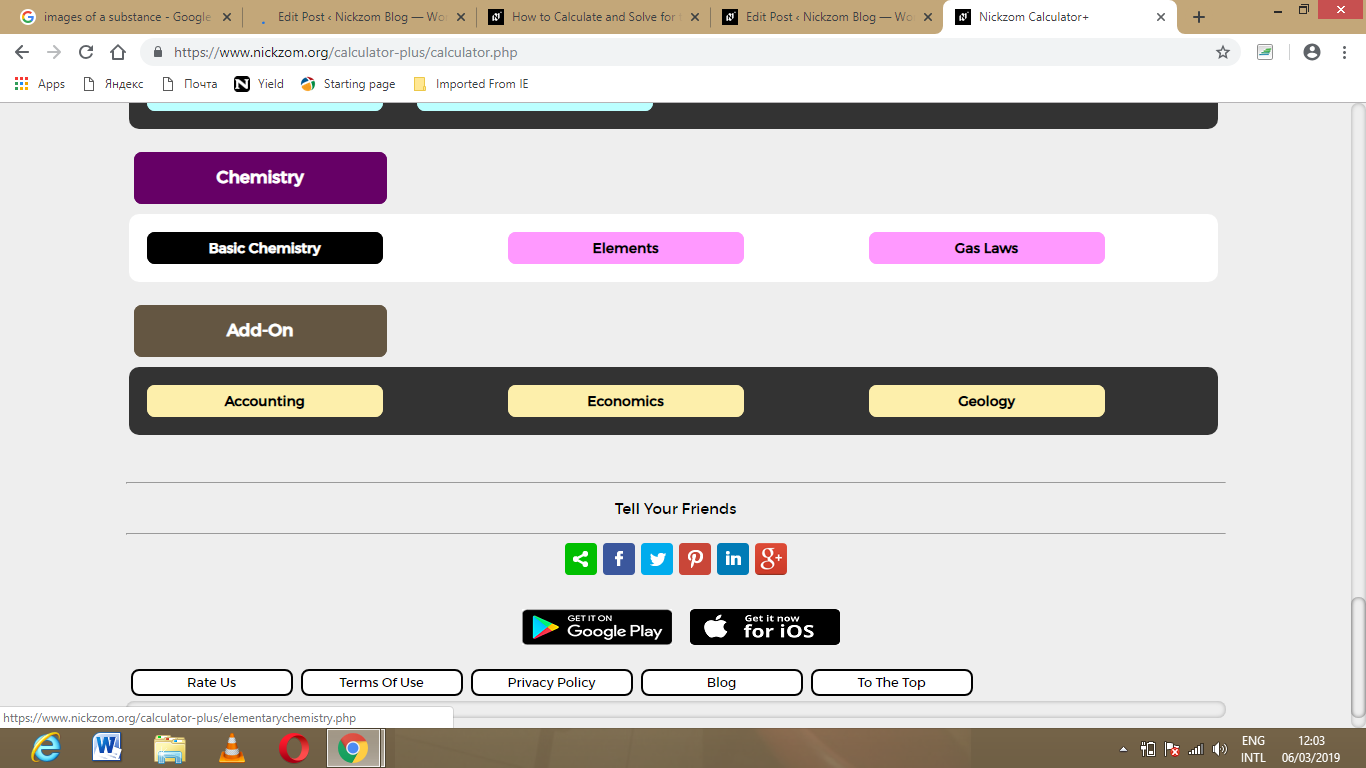Now, Click on Mass Concentration under Basic Chemistry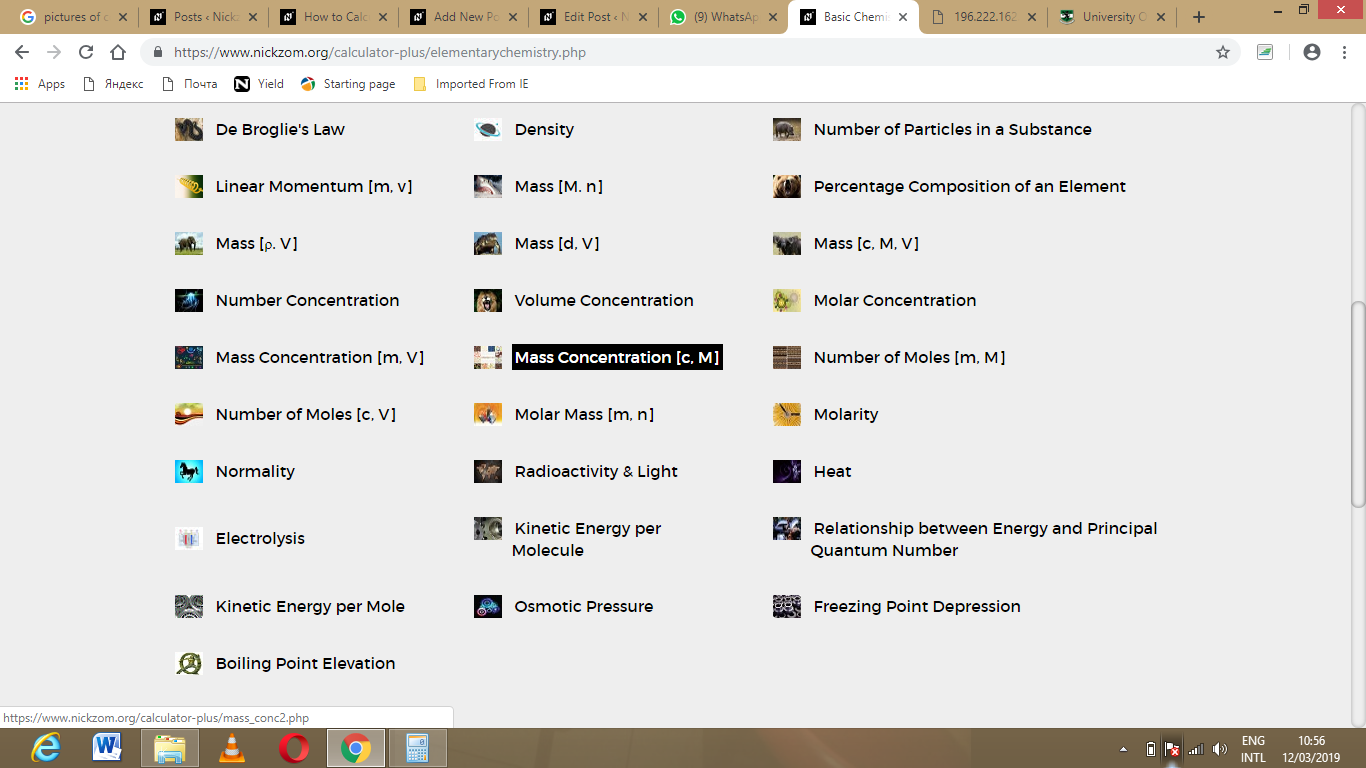The screenshot below displays the page or activity to enter your values, to get the answer for the mass concentration according to the respective parameters which are the molar concentration (c) and molar mass (M).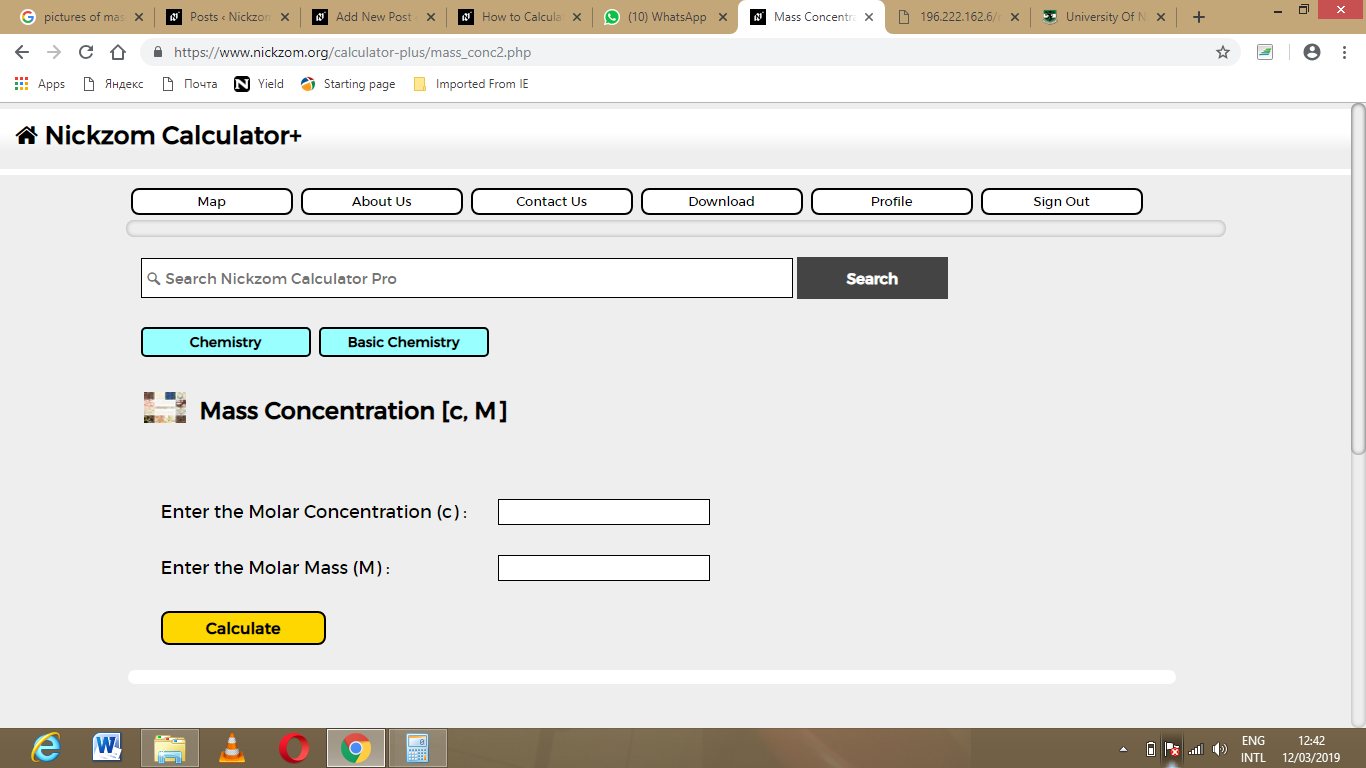Now, enter the values appropriately and accordingly for the parameters as required by the example above where the molar concentration (c) is 24 and molar mass (M) is 15.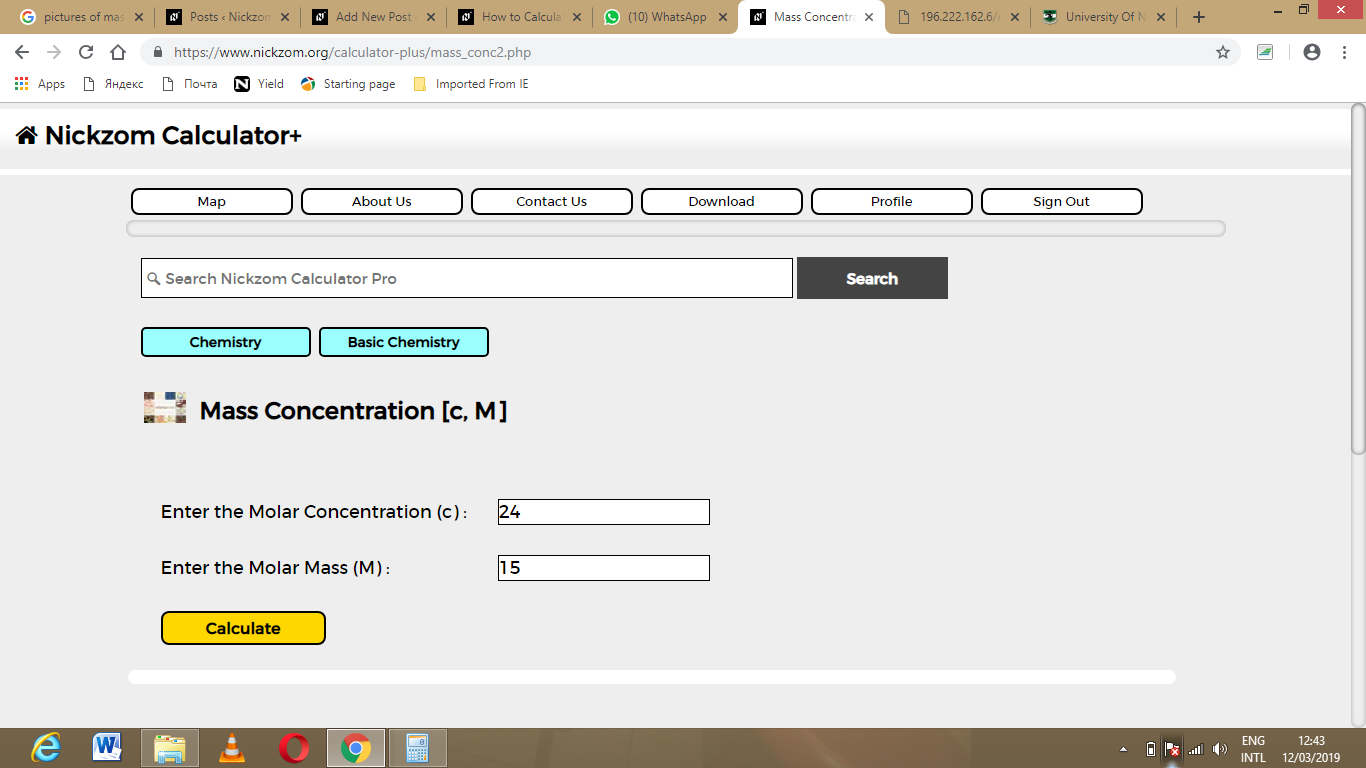Finally, Click on Calculate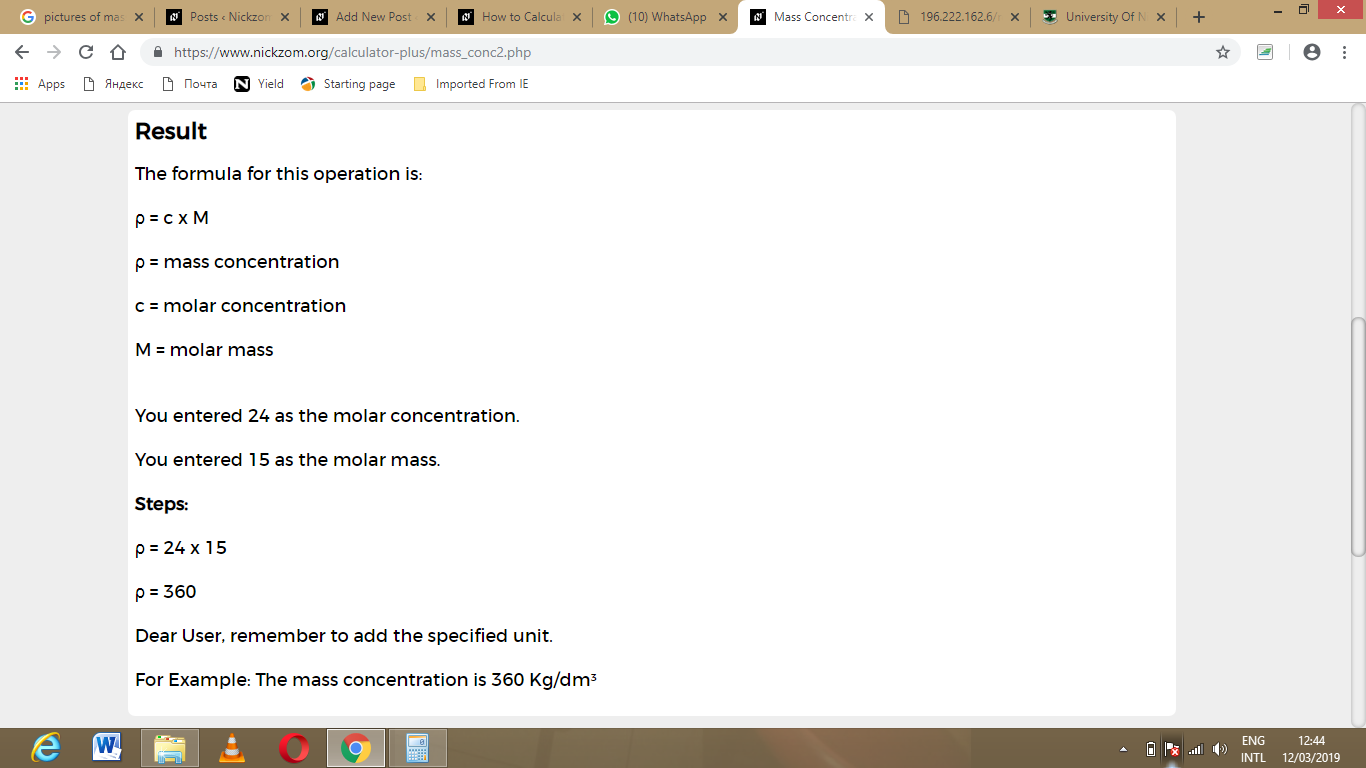As you can see from the screenshot above, Nickzom Calculator – The Calculator Encyclopedia solves for the mass concentration and presents the formula, workings and steps too.